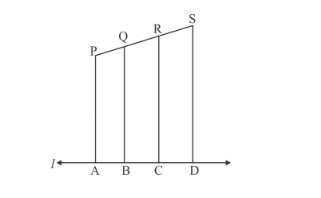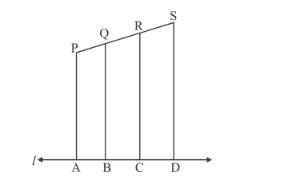# In the given figure, each of PA, QB, RC and SD is perpendicular to l.

Question:

In the given figure, each of PA, QB, RC and SD is perpendicular to l. If AB = 6 cm, BC = 9 cm, CD = 12 cm and PS = 36 cm, then determine PQ, QR and RS.Solution:

Given $A B=6 \mathrm{~cm}, B C=9 \mathrm{~cm}, C D=12 \mathrm{~cm}, A D=27 \mathrm{~cm}$ and $P S=36 \mathrm{~cm}$

PAQB, RC and SD is perpendicular to l,Therefore, by the corollory of basic proportionality theorem, we have

$\frac{A B}{A D}=\frac{P Q}{P S}$

$\frac{B C}{A D}=\frac{Q R}{P S}$

$\frac{C D}{A D}=\frac{R S}{P S}$

$\Rightarrow \frac{A B}{A D}=\frac{P Q}{P S}$

$\frac{6}{27}=\frac{P Q}{36}$

$\frac{6 \times 36}{27}=P Q$

$P Q=8$

Now for QR

$\frac{B C}{A D}=\frac{Q R}{P S}$

$\frac{9}{27}=\frac{Q R}{36}$

$\frac{9 \times 36}{27}=Q R$

$Q R=12$

Again for RS

$\frac{C D}{A D}=\frac{R S}{P S}$

$\frac{12}{27}=\frac{R S}{36}$

$\frac{12 \times 36}{27}=R S$

$R S=16$

Hence, the values of $P Q, Q R$ and $R S$ are $8,12,16$ respectively.